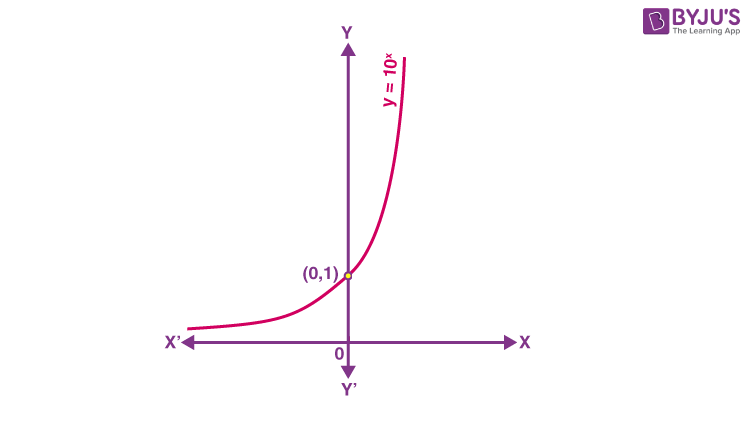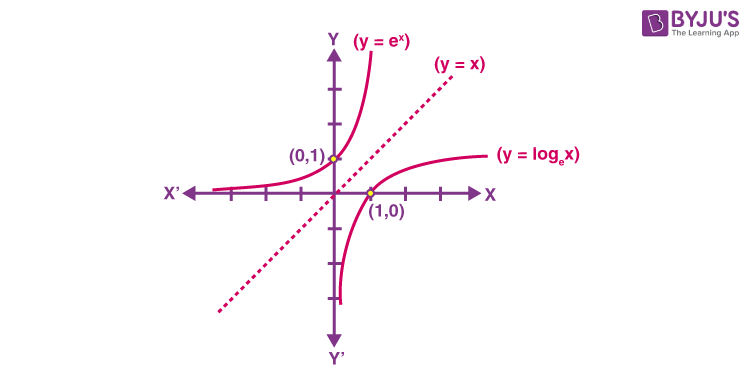# Exponential and Logarithmic Functions

In mathematics, we might have come across different types of functions such as polynomial functions, even functions, odd functions, rational functions and trigonometric functions, etc. In this article, you will learn about a new classification of functions called exponential functions and logarithmic functions.

## What are Exponential and Logarithmic Functions?

Exponential Function Definition:

An exponential function is a Mathematical function in the form y = f(x) = bx, where “x” is a variable and “b” is a constant which is called the base of the function such that b > 1. The most commonly used exponential function base is the transcendental number e, and the value of e is equal to 2.71828.

Using the base as “e” we can represent the exponential function as y = ex. This is called the natural exponential function. However, an exponential function with base 10 is called the common exponential function.

Logarithmic Function Definition:

If the inverse of the exponential function exists then we can represent the logarithmic function as given below:

Suppose b > 1 is a real number such that the logarithm of a to base b is x if bx = a.

The logarithm of a to base b can be written as logb a.

Thus, logb a = x if bx = a.

In other words, mathematically, by making a base b > 1, we may recognise logarithm as a function from positive real numbers to all real numbers. This function is known as the logarithmic function and is defined by:

logb : R+ → R

x → logb x = y if by = x

If the base b = 10, then it is called a common logarithm and if b = e, then it is called the natural logarithm. Generally, the natural logarithm is denoted by ln.

## Properties of Exponential and Logarithmic Functions

Some of the prominent features of the exponential functions are listed below:

• The domain of the exponential function is the set of all real numbers, i.e. R.
• The range of the exponential function is the set of all positive real numbers.
• The point (0, 1) is always on the graph of the given exponential function since it supports the fact that b0 = 1 for any real number b > 1.
• The exponential function is ever increasing; i.e., as we move from left to right, the graph rises above.
• For the large set of negative values of x, the exponential function is very close to 0; for example, the graph approaches the x-axis but never meets it.

These can be observed from the graph of an exponential function given below:Some of the essential considerations on the logarithm function to any base b > 1 are listed below:

• It is not possible to derive a meaningful definition of the logarithm for non-positive numbers, i.e. for negative numbers. So, the domain of the log function is the set of positive real numbers, i.e. R+.
• The range of the log function is the set of all real numbers.
• The point (1, 0) is always on the graph of the log function.
• The log function is ever-increasing, i.e., as we move from left to right the graph rises above.
• For the value of x quite near to zero, the value of log x can be made lesser than any given real number. That means, in quadrant IV, the graph approaches the y-axis but never meets it.In the above graph of y = ex and y = ln x, we observe that the two curves are the mirror images of each other reflected over the line y = x.

### Rules of Exponential and Logarithmic Functions

Below are the rules of exponential functions and logarithmic functions.

 Exponential Rules Logarithmic Rules ax ay = ax+y ax/ay = ax-y (ax)y = axy Axbx = (ab)x (a/b)x = ax/bx a0 = 1 a-x = 1/ ax logb (xy) = logb x + logb y logb (x/y) = logb x – logb y logb xm = m logb x logb p2 = logb p + logb p = 2 log p loga p = (logb p)/ (logb a) logb 1 = 0 logb b = 1 logb bx = x

### Exponential and Logarithmic Functions derivatives

The derivatives of exponential and logarithmic functions formulas are given below.

The derivative of ex with respect to x is written as:

$$\frac{d}{dx}(e^{x})=e^x$$

The derivative of log x with respect to x is written as:

$$\frac{d}{dx}(log\ x)=\frac{1}{x}$$

Using these two formulas, we can derive other formulas by applying exponential and logarithmic rules.

• The derivative of eax with respect to x is: $$\frac{d}{dx}(e^{ax})=ae^{ax}$$
• The nth derivative of eax with respect to x is: $$\frac{d^n}{dx}(e^{ax})=a^ne^{ax}$$

Similarly, we can derive multiple formulas for the derivative of exponential functions.

### Exponential and Logarithmic Functions Examples

Example 1:

Find the derivative of log (log x), x > 1, with respect to x.

Solution:

$$\frac{d}{dx}y = \frac{d}{dx}log(log\ x)$$

Using the formula d/dx (log x) = 1/x,

$$=\frac{1}{log\ x}\ \frac{d}{dx}(log\ x)$$ $$=\frac{1}{log\ x}. \frac{1}{x}$$

Therefore, (d/dx) [log (log x)] = 1/(x log x).

Example 2:

Simplify: y = 135/133

Solution:

y = 135/133

Using the quotient rule,

y = 135/133 = 13(5-3)

= 132

= 169

Example 3:

Evaluate the derivative of the function $$y=e^{x^4}$$.

Solution:

Given function is:

$$y=e^{x^4}$$

Differentiating with respect to x,

$$\frac{dy}{dx}=\frac{d}{dx}(e^{x^4}) = e^{x^4}\frac{d}{dx}(x^4)=e^{x^4}.4x^{4-1}=4x^3e^{x^4}$$

For more interesting concepts of mathematics, download BYJU’S – The Learning App today!